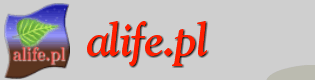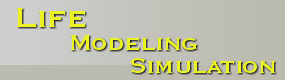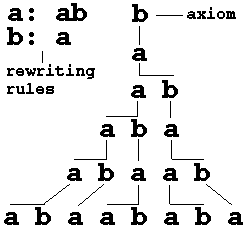# L-systems: a turtle draws plants

Lindenmayer systems – or L-systems for short – find an increasing number of applications in computer graphics, especially in fractals and realistic modelling of plants. More exotic applications, ranging from the reproduction of a traditional East Indian art form to graphically motivated algorithms for music composition, are also known.

L-systems base on productions – rules represented by strings. These rules define graphical structures.

Let us consider strings built of two letters a and b (they may occur many times in a string). For each letter we specify a rewriting rule. The rule a: ab means that the letter a is to be replaced by the string ab, and the rule b: a means that the letter b is to be replaced by a. The rewriting process starts from a distinguished string called the axiom. Let us assume that it consists of a single letter b. In the first derivation step (the first step of rewriting) the axiom b is replaced by a using production b: a. In the second step a is replaced by ab using production a: ab. The word ab consists of two letters, both of which are simultaneously replaced in the next derivation step. Thus, a is replaced by ab, b is replaced by a, and the string aba results. In a similar way (by the simultaneous replacement of all letters), the string aba yields abaab which in turn yields abaababa, then abaababaabaab, and so on (Figure 1).Figure 1. Example of a derivation in an L-system

Many fractals are thought of as sequence of primtive elements – line segments. The lengths of segments and the angles between them play a crucial role. To produce fractals, strings generated by L-systems must contain the necessary information about figure geometry. LOGO-style helps at defining graphical structures.
Firts of all we need to specify the angle increment so that the turtle will rotate by this angle when necessery. Step size defines the length of a single line. Given the step size and angle increment, the turtle can respond to commands represented by the following symbols:

F move forward a step of length d.
f move forward a step of length d without drawing a line.
+ turn right by angle increment
- turn left by angle increment
[ push the current state of the turtle onto a pushdown stack. The information saved on the stack contains the turtle's position and orientation, as well as the attributes such as the color and width of lines being drawn.
] pop a state from the stack and make it the current state of the turtle. No line is drawn, although in general the position of the turtle changes All others symbols are ignored by the turtle.

For instance the following L-system generates "quadratric Koch island" (see the example in the included applet):

F+F+F+F
F: F+F-F-FF+F+F-F
Having the recursion depth equal 1, L-system will produce a square. The higher the recursion depth set, the more sophisticated figure is produced.

Using stack symbols: [ and ] we can construct plan-like structures. Productions of such L-system would be responsible for creating apex, branches and leaves.